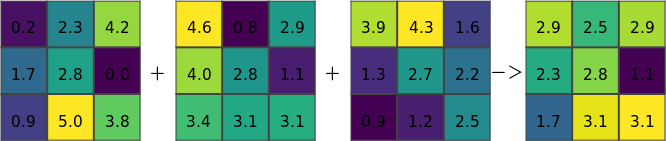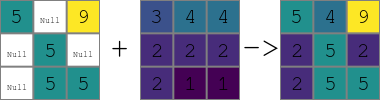## NAME

d.explanation.plot - Draw a plot of multiple rasters to explain a raster operation for example a + b = c

## KEYWORDS

display, manual, raster

## SYNOPSIS

d.explanation.plot
d.explanation.plot --help
d.explanation.plot a=name [b=name] [c=name] [d=name] [raster_font=string] [operator_ab=string] [operator_bc=string] [operator_cd=string] [operator_font=string] [label_a=string] [label_b=string] [label_c=string] [label_d=string] [label_font=string] [label_size=float] [bottom=float] [--help] [--verbose] [--quiet] [--ui]

### Flags:

--help
Print usage summary
--verbose
Verbose module output
--quiet
Quiet module output
--ui
Force launching GUI dialog

### Parameters:

a=name [required]
Name of input raster map
b=name
Name of input raster map
c=name
Name of input raster map
d=name
Name of input raster map
raster_font=string
Font for raster numbers
operator_ab=string
Operator between a and b
operator_bc=string
Operator between b and c
operator_cd=string
Operator between c and d
operator_font=string
Font for operators
label_a=string
Label above the raster
label_b=string
Label above the raster
label_c=string
Label above the raster
label_d=string
Label above the raster
label_font=string
Font for labels
label_size=float
Text size for labels
bottom=float
Offset from the bottom (percentage)

## DESCRIPTION

d.explantion.plot creates a plot of rasters and their relations which can serve as an explanation of a raster operation performed by a module or function.

Up to four rasters are supported. The default operators assume rasters to have the following relation:

a + b -> c

## EXAMPLES

### Example using generated data

In Bash:
```g.region n=99 s=0 e=99 w=0 rows=3 cols=3
r.mapcalc expression="a = rand(0., 5)" seed=1
r.mapcalc expression="b = rand(0., 5)" seed=2
r.mapcalc expression="c = rand(0., 5)" seed=3
r.series input=a,b,c output=d method=average
```
In Python:
```import grass.jupyter as gj
plot = gj.Map(use_region=True, width=700, height=700)
plot.d_background(color="white")
plot.run("d.explanation.plot", a="a", b="b", c="c", d="d", operator_font="FreeMono:Regular")
plot.show()
```Figure: Resulting image for r.series

### Example using artificial data

```r.in.ascii input=- output=input_1 <<EOF
north: 103
south: 100
east: 103
west: 100
rows: 3
cols: 3
5 * 9
* 5 *
* 5 5
EOF
r.in.ascii input=- output=input_2 <<EOF
north: 103
south: 100
east: 103
west: 100
rows: 3
cols: 3
3 4 4
2 2 2
2 1 1
EOF
r.colors map=input_1,input_2 color=viridis
g.region raster=input_1
r.patch input=input_1,input_2 output=result
d.mon wx0 width=400 height=400 output=r_patch.png
d.explanation.plot a=input_1 b=input_2 c=result
```Figure: Resulting image for r.patch

## KNOWN ISSUES

• Issue #3381 prevents d.rast.num to be used with d.mon cairo, so d.mon wx0 needs to be used with this module. Using environmental variables for rendering directly or using tools such as Map from grass.jupyter avoids the issues.
• Issue #3382 prevents usage of centered text with d.mon wx0, so the hardcoded values for text does not work perfectly.
• Issue #3383 prevents d.rast.num to be saved to the image with d.mon wx0, taking screenshot is necessary (with a powerful screenshot tool, this also addresses the copping issue below).
• The size of the display must be square to have rasters and their cells as squares, e.g., d.mon wx0 width=400 height=400 must be used. The image needs to be cropped afterwards, e.g. using ImageMagic's mogrify -trim image.png.

g.region, d.frame, d.rast.num, d.grid, d.mon, v.mkgrid

## AUTHOR

Vaclav Petras, NCSU GeoForAll Lab

## SOURCE CODE

Available at: d.explanation.plot source code (history)

Latest change: Tuesday Oct 04 23:05:29 2022 in commit: b4cfa50ee83494c188c024548a4ff4f0600dc551

© 2003-2023 GRASS Development Team, GRASS GIS 8.3.1dev Reference Manual